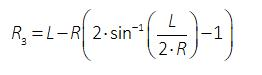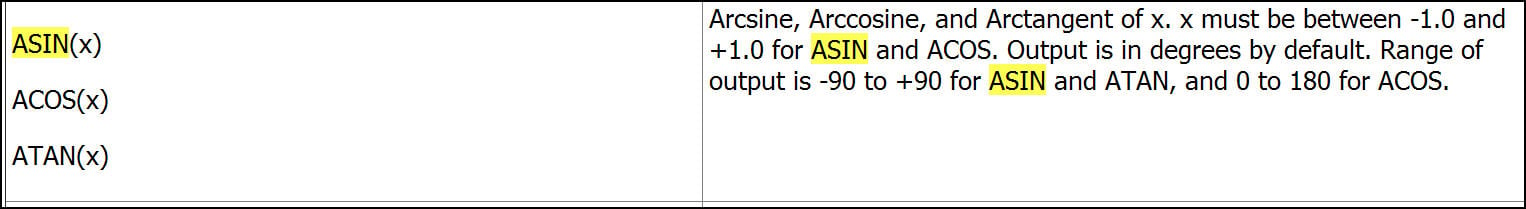## 3D Design

•jonsys
Subscriber

Because of parametrizing my CAD geometry in DesignModeler, I am using formulas. Under Parameters>Parameter/Dimension Assignments I want to apply this formula for one of the variables:L and R are known and represent lengths [mm]. For sin^-1 I use the asin(arg) function. I want to get the asin(arg) value in radians but I guess something is being messed up with degrees.

That is how the expression looks like right now: @L-@R*(2*asin(@L/2/R)-1)

How should I write it?

•peteroznewman
Subscriber

The output of asin is in degrees, so just divide by 57.29578 to convert to radians.

•Keyur Kanade
Ansys Employee

Hi,

Arcsine, Arccosine, and Arctangent of x. x must be between -1.0 and +1.0 for ASIN and ACOS. Output is in degrees by default. Range of output is -90 to +90 for ASIN and ATAN, and 0 to 180 for ACOS. See image from help.Hope this helps.

Regards,

Keyur

•jonsys
Subscriber

•peteroznewman